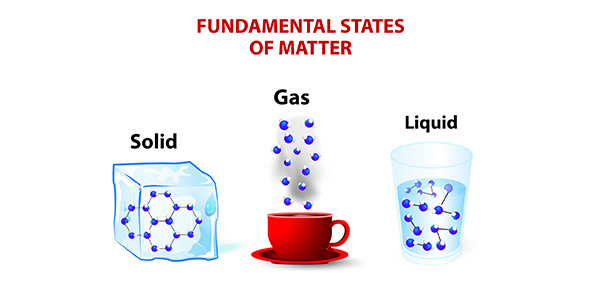# States Of Matter

10 QuestionsSettingsStudents will take an assessment on what they have learned about the states of matter.

Related Topics
• 1.
How many states are there of matter?
• A.

4

• B.

2

• C.

3

• D.

5

• 2.
What are the states of Matter?
• A.

Solid, Liquid, Gas

• B.

Light, Water, Carbon

• C.

Energy, Water

• D.

Atoms, Molecules

• 3.
Gas assumes the shape and the volume of its container.
• A.

True

• B.

False

• 4.
Retains a fixed volume and shape
• 5.
Liquids and solids flow easily, while gases doesn't flow as easy.
• A.

False

• B.

True

• 6.
The particles of a gas are packed tightly together
• A.

True

• B.

False

• 7.
When a solid changes to a liquid it is called what?
• A.

Melting

• B.

Water

• C.

Freezing

• D.

Juice

• 8.
What state can fit into any container of any size and any shape?
• A.

Solid

• B.

Energy

• C.

Plasma

• D.

Liquid

• 9.
Which statement is true?
• A.

A solid can become a liquid.

• B.

A solid can not become a liquid.

• C.

A gas can become a liquid.

• D.

A liquid can not become a solid.

• 10.
Liquid assumes the shape of the part of the container which it occupies
• A.

True

• B.

False